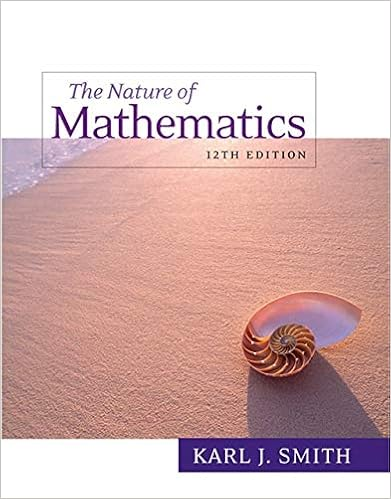# week7 - Math 31B Spring 14 WEEK 7 Part 1 Taylors theorem It...

• Notes
• 5

This preview shows page 1 - 3 out of 5 pages.

##### We have textbook solutions for you!
The document you are viewing contains questions related to this textbook.The document you are viewing contains questions related to this textbook.
Chapter 18 / Exercise 5
Nature of Mathematics
SmithExpert Verified
Math 31B. Spring ’14. WEEK 7. Part 1. Taylor’s theorem: It gives a way of approxmating a function by a polynomial f ( x ) = f ( a ) + f 0 ( a )( x - a ) + 1 2 f 00 ( a )( x - a ) 2 + . . . + 1 k ! f ( k ) ( a )( x - a ) k + . . . + 1 N ! f ( N ) ( a )( x - a ) N + R N ( f, x, a ) Here f ( k ) ( a ) represents the value at a of the k -th derivative of f . The quantity R N ( f, x, a ) is very small in the sense that it approximates zero faster than ( x - a ) N , in the sense that lim x ! a R N ( f, x, a ) ( x - a ) N = 0 .
##### We have textbook solutions for you!
The document you are viewing contains questions related to this textbook.The document you are viewing contains questions related to this textbook.
Chapter 18 / Exercise 5
Nature of Mathematics
SmithExpert Verified
Since f 0 ( x ) = e x , then f ( k ) ( x ) = e x always, thus f ( k ) (0) = 1 for k = 1 , 2 , 3 , . . . . Using the definition of
•••